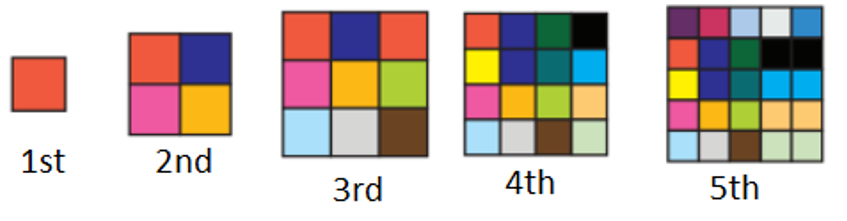Mathematics
Easy

Question

# Joshua made a pattern using square tiles.If the pattern continues, the number of square tiles required to make the 9th shape is

## 79    72    81    108Hint:

## The correct answer is: 81

### The number of tiles in first shape is 1, second shape is 4, third shape is fourth shape is 16 and fifth shape is 25.IIf we see there is a relation between the number of shape and number of tiles. The number of tiles is the square of the number of shape.Therefore, the rule used is “ the square of number of shapes”.First shape has 12 = 1 tile.Second shape has 22 = 4 tiles.Third shape has 32 = 9 tiles.Fourth shape has 42 = 16 tiles.Fifth shape has 52 = 25 tiles.We are asked to find number of tiles in 9th shape.Ninth shape will have 92 = 81 shapes.The number of tiles in 9th shape are 81.

For such questions, the main part is to find the rule used to form the pattern.

### Related Questions to study#### With Turito Foundation.#### Get an Expert Advice From Turito.